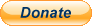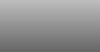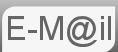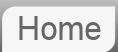# Answer to Puzzle #14: 52 Cards Win a Dollar

14. You have 52 playing cards (26 red, 26 black). You draw cards one by one. A red card pays you a dollar. A black one fines you a dollar. You can stop any time you want. Cards are not returned to the deck after being drawn. What is the optimal stopping rule in terms of maximizing expected payoff?

Also, what is the expected payoff following this optimal rule?

The solution to this problem is, in my opinion the most difficult to understand of all the puzzles. Indeed I was unable to solve it and didn't receive a complete solution until two years after originally posting it. The final solution, in the form of the spreadsheet was sent to me by Han Zheng.

For this reason I have left on the page the thoughts I had before I had the final solution as they represent an easier to understand and more simplistic approach. Also the reasoning may help you arrive at the final solution by yourself or help you understand it. I would recommend reading that answer before you dive into the full answer. But an important thing to note is that as the player we can't lose this game as we can gamble till all the cards are drawn and our net position is zero.

From our earlier analysis it is clear we need a dynamic quit rule. A signal value, (we will quit when we are x dollars ahead,) is not sufficient. We must, at each stage, consider what cards are remaining, and therefore the probability of a positive or negative outcome from drawing again.

What we are about to do is attempt to compare, at any point in the game, the cash we are holding against the mathematically expected return of gambling. Simply if we are holding more cash than the expected return of gambling we will stop. The mathematically expected return will be calculated by the weighted probability of either outcome of the gamble and those positions' expected returns. Which, in turn, will be calculated from their possible outcomes and their probabilities, and so on.

For the explanation I will ask you first to consider a deck containing only 6 cards, 3 +ve & 3 -ve (note I'm no longer calling the cards black and red, it confuses me.)
 Negative Cards Remaining (N) 3 2 1 0 +ve Cards remaining (P) 3 .5 0 .5 .4 -1 .6 .25 -2 .75 0 -3 1 2 .6 1 .4 .5 0 .5 1/3 -1 2/3 0 -2 1 1 .75 2 .25 2/3 1 1/3 .5 0 .5 0 -1 1 0 1 3 0 1 2 0 1 1 0 0

Things to note about the table:
• We start the game with 3 of each card remaining ie the top left
• The number in black represents our cash position at that point in the game
• The red number represents the fractional chance of us moving right ie drawing a -ve card
• The number in green represents the fractional chance of us moving down ie drawing a +ve card
Let N & P be the numbers of Negative & Positive cards remaining respectively.

The probability of moving down is given by P/(N+P), that is to say the Positive cards remaining divided by (N+P), the total number of cards remaining. The probability of moving right is N/(N+P) and the cash value of a cell is N-P. N-P because the mumber of Positive cards drawn is 3-P, the cash value is then (3- P)-(3-N)

There are some cells we can intuitively speculate as to our course of action without too much trouble:

If we are in cell 3,0 (ie 3 -ve remaining and 0 +ve remaining, cell looks like 1 3 0) we have 100% chance of negatively affecting our position so we "stick"

In cell 0,1 (no Negative cards remaining, 1 Positive, cell looks like 0 -1 1) we have 100% chance of improving our position, indeed this is true of all the cells in the 0 column

Now consider the cell 1,1 (as an example,) from here we have a 50% chance of improving our position to +1 (moving down,) and a 50% chance of harming it to -1. So the weighted outcome of a gamble might appear to be even. However, if we were to win from this gamble we would quit on +1 in cell 1,0, but if we were to lose this gamble we would "gamble" again from cell 0,1 to a cash position of zero.

This means we can consider the expected return from cell 0,1 to be 0 and from 1,0 to be 1. More formally, for those interested in a more mathematical approach the expected return for N=0 is zero and for P=0 is N

To calculate the expected return from 1,1 we then have 50% chance of 0 plus 50% chance of 1 ie 0.5.

That is to say the expected return, with one of each card remaining is 0.5 which is an improvement on our cash position of zero.

We can now calculate the expected return from 2,1 (cell looks like 2/3 1 1/3,) it's 2/3 moving right (harming) plus 1/3 moving down (improving) or 2/3 of cell 1,1 plus 1/3 of cell 2,0 which calculates as (2/3)*.5 + (1/3)*2 = 1

(note we used the expected return for (1,1) that we calculated earlier.)

Using the same procedure and our expected return from 2,1 (of value 1,) we can do the same for cell 3,1 (cell looks like .75 2 .25)

Expected return is given by .25 * cell(3,0) + .75 * cell(2,1) = .25 * 3 + .75 * 1 = 1.5

So We have that the expected return from cell 3,1 is 1.5. But in cell 3,1 we are holding 2 dollars in our hand, so gambling would not be in our interest, as it would harm our expected return.

This is our quit rule!!!!

We quit when the mathematical expected return of gambling is less than the cash we are currently holding.

We calculate the expected return of gambling as being the probability weighted outcome of the winning and losing cells, where the value assigned to these cells is in-turn their expected return.

This approach can be extended to a 52 card deck.

The spread sheet below does this calculation, the value in each cell is the maximum of either what we are currently holding or the expected return of a gamble. If the former is the maximum then we quit..... For the avoidance of doubt, each cell shows the expected return from the position represented by that cell. So at the beginning of the game the expected return is \$2.624475549

Ben Howe has written a paper on this problem, Red Black Card Game and Generalized Catalan Numbers is a rigorous statistical approach beyond the scope of this site.

## My original Solution...

Firstly I must concede I have nothing approaching a complete solution for this, I've consulted many Mathematicians and am still working on a complete solution. However, i have been getting e-mails about this, so i thought I'd give you what i have so far...

## What do we mean by a stopping rule????

A Stopping rule could be anything, it could be 'I'll stop after just one game', 'I'll wont play the game...' etc...

In this section we will consider the stopping rule:

I will quit when I get 'x' Dollars ahead.

Where we will have to determine x.

## The first thing to note:

We can't lose!!!!! Since there are 26 black cards and 26 red if we are behind we can just keep playing until the end and we will be even, not up, not down.

My first thought was that a good quit strategy given this fact would be to simply quit when we get ahead. This however does not yield the maximum profit.

That is to say x is not equal to 1. Why? Well it is true that whenever we are \$1 up necessarily the cards become stacked against us, we are more likely to lose the next gamble. But the most we can lose is \$1 since we can play to zero. On the other hand if we continue we could go on to win \$3, \$4, \$5 etc. So well the odds are against us winning the upside is much greater than the downside.

The tool below will run a Monte Carlo simulation in your browser to help determine the value of x with the highest expected return.

Note: no information is sent to me, the calculation is done entirely locally, on your computer.
Enter a quit value.
Number of times to run simulation.

This shows us the best return is when x or our quit value is 4. However there may be more we can do:

## Further thinking...

A clear limitation of our model is that, what I call the quit value, is static.

consider this:

We are using a value for x of 4, we have just drawn the 51st card, and we are one dollar ahead.

In this case we know that the next card will be such that we will lose our one dollar. so in this instance we should change our quit value to 1. Clearly this is the beginning of a model that allows us to change the value of depending on how the deck is loaded....

I will continue to play with this idea and would welcome any suggestions for a model along with their expected returns. I'll happily post the results here.

I think Bard had the best stab at this. But it did include the phrase 'the optimal stopping rule can be calculated using a computer program' which is odd given what it is.

If you're curious what Bard made of this puzzle...

If you're curious what ChatGPT made of this puzzle...

© Nigel Coldwell 2004 -  – The questions on this site may be reproduced without further permission, I do not claim copyright over them. The answers are mine and may not be reproduced without my expressed prior consent. Please inquire using the link at the top of the page. Secure version of this page.PayPal
I always think it's arrogant to add a donate button, but it has been requested. If I help you get a job though, you could buy me a pint! - nigel